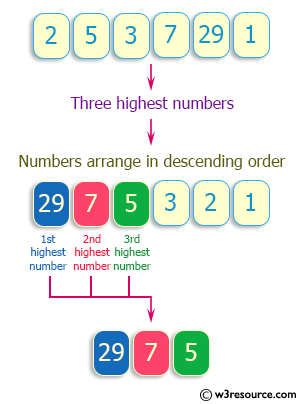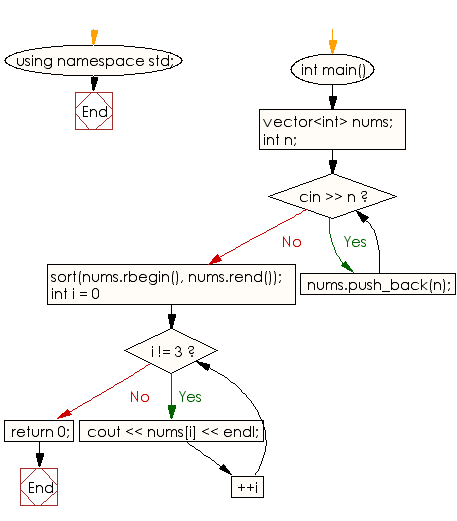﻿ C++ : Prints 3 highest numbers in descending order of a list

# C++ Exercises: Prints three highest numbers from a list of numbers in descending order

## C++ Basic: Exercise-63 with Solution

Write a C++ program that prints the three highest numbers from a list of numbers in descending order.

Pictorial Presentation:Sample Solution:

C++ Code :

``````#include <iostream>
#include <vector>
#include <algorithm>
using namespace std;
int main() {
vector<int> nums;
int n;
while (cin >> n) {
nums.push_back(n);
}
sort(nums.rbegin(), nums.rend());

for (int i = 0; i != 3; ++i) {
cout << nums[i] << endl;
}
return 0;
}
``````

Sample Output:

```Sample input : 2 5 3 7 29 1
Sample Output:
29
7
5
```

Flowchart:C++ Code Editor:

What is the difficulty level of this exercise?

﻿

## C++ Programming: Tips of the Day

Why is there no std::stou?

The most pat answer would be that the C library has no corresponding "strtou", and the C++11 string functions are all just thinly veiled wrappers around the C library functions: The std::sto* functions mirror strto*, and the std::to_string functions use sprintf.

Ref: https://bit.ly/3wtz2qA

We are closing our Disqus commenting system for some maintenanace issues. You may write to us at reach[at]yahoo[dot]com or visit us at Facebook